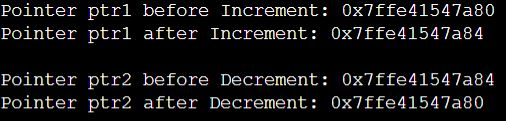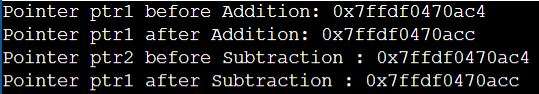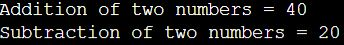# Arithmetic Operations on Pointers in C

## Arithmetic Operations on Pointers in C Language with Examples

In this article, I will discuss Arithmetic Operations on Pointers in C Language with Examples. Please read our previous articles discussing Pointers in C Language with Examples. At the end of this article, you will understand the different arithmetic operations we can perform on the pointer in C language.

##### Arithmetic Operations on Pointers in C:

As we already discussed in our previous article, the Pointer variable stores another variable’s address. The address is nothing but the memory location assigned to the variable. That means the pointer doesn’t store any value. It stores the address. Hence, only a few operations are allowed to be performed on Pointers. The operations are slightly different from those we generally use for mathematical calculations. The operations are as follows:

1. Increment/Decrement of a Pointer
2. Addition of integer to a pointer
3. Subtraction of integer to a pointer
4. Subtracting two pointers of the same type
##### Increment/Decrement Operation of a Pointer in C Language

Increment: When a pointer is incremented, it actually increments by the number equal to the size of the data type for which it is a pointer. For Example, If the pointer is of type integer and the pointer stores address 1000 and if the pointer is incremented, it will increment by 2(size of an int), and the new address that the pointer will point to is 1002. If the pointer is of type float and stores the address 1000, and if the pointer is incremented, it will increment by 4(size of a float), and the new address will be 1004.

Decrement: It is just the opposite of the Increment operation. When a pointer is decremented, it actually decrements by the number equal to the size of the data type for which it is a pointer. For Example, If the pointer is of type integer and if the pointer stores the address 1000, and if the pointer is decremented, then it will decrement by 2(size of an int), and the new address that the pointer will point to is 998. If the pointer is of type float and if it stores the address 1000, and if the pointer is decremented, then it will decrement by 4(size of a float), and the new address will be 996.

###### Program to Understand Increment/Decrement Operation of a Pointer in C

The following program illustrates pointer increment/decrement operation using C Language.

```#include <stdio.h>
int main ()
{
// Integer variable
int a = 10, b=20;

// Pointer to an integer
int *ptr1, *ptr2;

// Pointer stores the address of variable a
ptr1 = &a;
ptr2 = &b;

printf ("Pointer ptr1 before Increment: ");
printf ("%p \n", ptr1);

// Incrementing pointer ptr1;
ptr1++;

printf ("Pointer ptr1 after Increment: ");
printf ("%p \n\n", ptr1);

printf ("Pointer ptr2 before Decrement: ");
printf ("%p \n", ptr2);

// Decrementing pointer ptr2;
ptr2--;

printf ("Pointer ptr2 after Decrement: ");
printf ("%p \n\n", ptr2);

return 0;
}
```
###### Output:##### Addition and Subtraction Arithmetic Operation on Pointer in C Language

Let us see how to perform Addition and Subtraction Operations on a Pointer in C Language.

1. Addition: In C Programming Language, when a pointer is added with a value, the value is first multiplied by the size of the data type and then added to the pointer.
2. Subtraction: In C Programming Language, when a pointer is subtracted with a value, the value is first multiplied by the size of the data type and then subtracted from the pointer.

The following program illustrates Addition and Subtraction Arithmetic Operations on a Pointer in C Language.

```#include <stdio.h>
int main()
{
// Integer variable
int a = 10;

// Pointer to an integer
int *ptr1, *ptr2;

// Pointer stores the address of variable a
ptr1 = &a;
ptr2 = &a;

printf("%p \n", ptr1);

// Addition of 2 to pointer ptr1
ptr1 = ptr1 + 2;
printf("%p \n", ptr1);

printf("Pointer ptr2 before Subtraction : ");
printf("%p \n", ptr2);

// Subtraction of 2 from pointer ptr2
ptr2 = ptr2 + 2;
printf("Pointer ptr1 after Subtraction : ");
printf("%p \n", ptr2);
return 0;
}```
###### Output:##### Addition and Subtraction of Two Pointers in C Language

In the example below, we created two integer pointers and then performed the addition and subtraction operation.

```#include<stdio.h>
int main ()
{
int a = 30, b = 10, *p1, *p2, sum;
p1 = &a;
p2 = &b;
sum = *p1 + *p2;
printf ("Addition of two numbers = %d\n", sum);
sum = *p1 - *p2;
printf ("Subtraction of two numbers = %d\n", sum);
return 0;
}```
###### Output:##### Arithmetic Operations on Pointers in C

In C programming, pointers are variables that store the memory addresses of other variables. Arithmetic operations on pointers can be quite useful, especially in array manipulation and dynamic memory management. Let’s discuss the various arithmetic operations that can be performed on pointers:

• Pointer Increment (++): Incrementing a pointer advances it to point to the next element of its type. For example, if int *p points to an integer, p++ will increase p so it points to the next integer in memory (typically 4 bytes ahead on a system where int is 4 bytes).
• Pointer Decrement (–): Decrementing a pointer does the opposite of incrementing. It makes the pointer point to the previous element of its type.
• Pointer Addition: You can add an integer value to a pointer. If p is a pointer and n is an integer, p + n increments p to point to the nth element ahead. This does not change the value of p itself unless you assign the result to p.
• Pointer Subtraction: Subtracting an integer from a pointer, as in p – n, moves the pointer n elements back.
• Subtracting Two Pointers: When you subtract one pointer from another, as in p1 – p2, the result is the number of elements between the two pointers, assuming both pointers point to elements of the same array.
• Comparing Pointers: Pointers can be compared using relational operators like ==, !=, >, <, >=, and <=. This can be useful to check if two pointers point to the same location or to determine the relative positions of pointers in the same array.

Let’s go through some examples of arithmetic operations using pointers in C. These examples will help illustrate how pointer arithmetic works in different scenarios.

###### Example 1: Pointer Increment
```#include <stdio.h>

int main() {
int arr[] = {10, 20, 30, 40, 50};
int *p = arr;  // Pointer to the first element of the array

printf("Original address: %p, Value: %d\n", (void*)p, *p);
p++;  // Increment the pointer
printf("New address: %p, Value: %d\n", (void*)p, *p);

return 0;
}
```

In this example, p initially points to the first element of arr. After the increment (p++), p points to the second element.

###### Example 2: Pointer Decrement
```#include <stdio.h>

int main() {
int arr[] = {10, 20, 30, 40, 50};
int *p = &arr;  // Pointer to the fourth element of the array

printf("Original address: %p, Value: %d\n", (void*)p, *p);
p--;  // Decrement the pointer
printf("New address: %p, Value: %d\n", (void*)p, *p);

return 0;
}```

Here, p is set to point to the fourth element of arr. After decrementing (p–), it points to the third element.

```#include <stdio.h>

int main() {
int arr[] = {10, 20, 30, 40, 50};
int *p = arr;  // Pointer to the first element

// Move the pointer 2 elements forward
p = p + 2;
printf("Address: %p, Value: %d\n", (void*)p, *p);

return 0;
}```

In this case, p + 2 moves the pointer p two elements forward, so it points to the third element (30).

###### Example 4: Pointer Subtraction
```#include <stdio.h>

int main() {
int arr[] = {10, 20, 30, 40, 50};
int *p = &arr;  // Pointer to the last element

// Move the pointer 2 elements back
p = p - 2;
printf("Address: %p, Value: %d\n", (void*)p, *p);

return 0;
}```

p – 2 moves the pointer p two elements back, pointing it to the third element from the last.

###### Example 5: Subtracting Two Pointers
```#include <stdio.h>

int main() {
int arr[] = {10, 20, 30, 40, 50};
int *p1 = &arr;  // Pointer to the last element
int *p2 = arr;      // Pointer to the first element

int diff = p1 - p2;
printf("Difference in elements: %d\n", diff);

return 0;
}```

This example calculates the difference between two pointers, p1 and p2, which is the number of elements between them in the array.